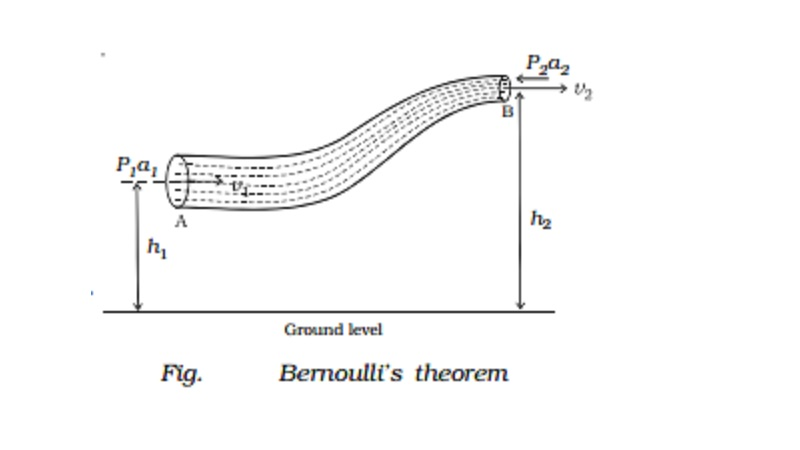Home | | Physics | | Physics | Bernoulli's theorem

# Bernoulli's theoremAccording to Bernoulli's theorem, for the streamline flow of a non-viscous and incompressible liquid, the sum of the pressure energy, kinetic energy and potential energy per unit mass is a constant.

Bernoulli's theorem

In 1738, Daniel Bernoulli proposed a theorem for the streamline flow of a liquid based on the law of conservation of energy. According to Bernoulli's theorem, for the streamline flow of a non-viscous and incompressible liquid, the sum of the pressure energy, kinetic energy and potential energy per unit mass is a constant.

P/p + v2 +gh = constant.

This equation is known as Bernoulli's equation.

Consider streamline flow of a liquid of density through a pipe AB of varying cross section. Let P1 and P2 be the pressures and a1 and a2, the cross sectional areas at A and B respectively. The liquid enters A normally with a velocity v1 and leaves B normally with a velocity v2. The liquid is accelerated against the force of gravity while flowing from A to B, because the height of B is greater than that of A from the ground level. Therefore P1 is greater than P2. This is maintained by an external force.

The mass m of the liquid crossing per second through any section of the tube in accordance with the equation of continuity is

a1v1ρ = a2v2ρ = m

or

a1v1 = a2v2 = m/p =V

As a1 > a2 , v1 < v2

The force acting on the liquid at A = P1a1

The force acting on the liquid at B = P2 a2

Work done per second on the liquid at A = P1a1 � v1 = P1V

Work done by the liquid at B = P2a2 � v2 = P2V

Net work done per second on the liquid by the pressure energy

in moving the liquid from A to B is = P1V - P2V ...(2)

If the mass of the liquid flowing in one second from A to B is m, then increase in potential energy per second of liquid from A to B is mgh2 - mgh1

Increase in kinetic energy per second of the liquid

= � mv22 - � mv12

According to work-energy principle, work done per second by the pressure energy = Increase in potential energy per second + Increase in kinetic energy per second

P/p + gh + � v2 = constant

This is Bernoulli's equation. Thus the total energy of unit mass of liquid remains constant.

Dividing equation (3) by g, p/pg + v2/2g + h = constant

Each term in this equation has the dimension of length and hence is called head. p/pg is called pressure head, v2/2g is velocity head and h is the gravitational head.

Special case :

If the liquid flows through a horizontal tube, h1 = h2. Therefore there is no increase in potential energy of the liquid i.e. the gravitational head becomes zero.

equation (3) becomes

P/p + 1/2v2 = a constant

This is another form of Bernoulli's equation.

Application of Bernoulli's theorem

(i) Lift of an aircraft wing

A section of an aircraft wing and the flow lines are shown in Fig. The orientation of the wing relative to the flow direction causes the flow lines to crowd together above the wing. This corresponds to increased velocity in this region and  hence the pressure is reduced. But below the wing, the pressure is nearly equal to the atmospheric pressure. As a result of this, the upward force on the underside of the wing is greater than the downward force on the topside. Thus there is a net upward force or lift.

(ii) Blowing of roofs

During a storm, the roofs of huts or tinned roofs are blown off without any damage to other parts of the hut. The blowing wind creates a low pressure P1 on top of the roof. The pressure P2 under the roof is however greater than P1. Due to this pressure difference, the roof is lifted  and blown off with the wind.

(iii) Bunsen burner

In a Bunsen burner, the gas comes out of the nozzle with high velocity. Due to this the pressure in the stem of the burner decreases. So, air from the atmosphere rushes into the burner.

(iv) Motion of two parallel boats

When two boats separated by a small distance row parallel to each other along the same direction, the velocity of water between the boats becomes very large compared to that on the outer sides. Because of this, the pressure in between the two boats gets reduced. The high pressure on the outer side pushes the boats inwards. As a result of this, the boats come closer and may even collide.

Study Material, Lecturing Notes, Assignment, Reference, Wiki description explanation, brief detail

Related Topics